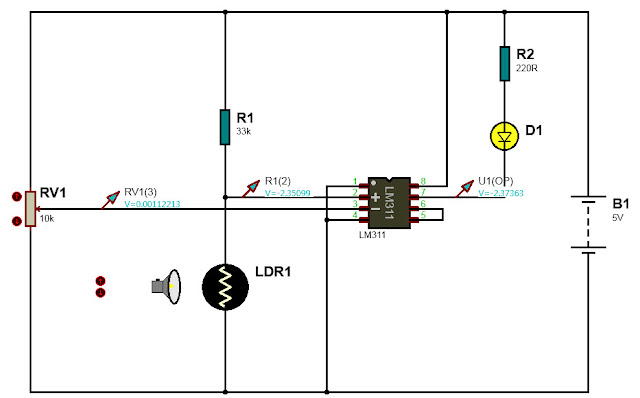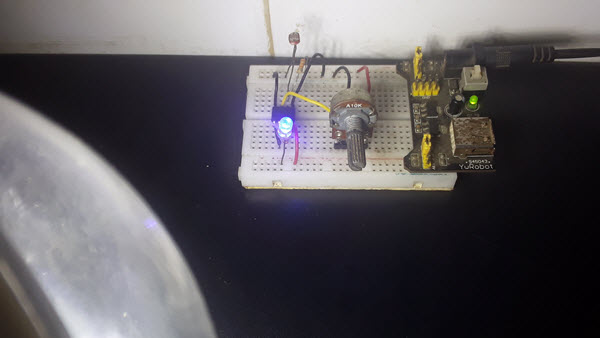# Light sensor using LDR and LM311

Here it is shown how one can build a light sensor detector using LM311 and LDR(Light Dependent Resistor). The light sensor detector uses Light Dependent Resistor(LDR) which is sensitive to light. When light shines on LDR, the resistance decreases. This light dependent resistance property can be used with the LM311 comparator to produce LOW voltage at the output if the input voltage

The following shows the light detector circuit diagram build with LDR and LM311.

Two voltage divider are connected to the non-inverting pin 2 and inverting pin 3. The voltage divider circuit connected to the +ve terminal is made using two resistors- 33KOhm and the LDR. The voltage divider connected to the -ve terminal is the 10KOhm potentiometer. The output of the  LM311 comparator is,

$V_{out} = V_+ - V_-$

As shown in the diagram we have a LED connected via 220Ohm resistor from the +5V to the LM311 output. The voltage across the LED is 2.2V.

In normal state when there is no light near the LDR, the voltage at the +ve terminal of LM311 is around 2.3V, the voltage at the -ve terminal is around 0.1V and so the output voltage at pin 7 is around 2.4V. Since the voltage at the output is 2.4V and the voltage at the anode is 2.4V the LED is turned off.

When light is shined on LDR, the voltage at the +ve terminal is around -2.4V and the voltage at the -ve terminal is around 0.1V and so the output is around -2.3V. In this case, since the output is -2.3V and the voltage at the anode is 2.4V current will flow across the LED and the LED is turned on.The light sensor build on breadboard is shown below.In this tutorial we show how LM311 and LDR can be used to make a light sensor detector. See other light sensor tutorials below.